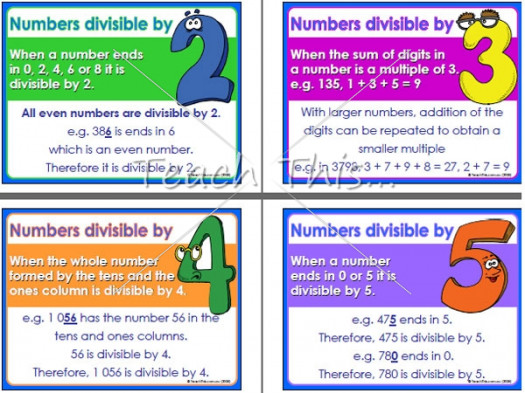# What Do You Know About Inequalities: Greater Than And Less Than Basics?

10 Questions | Total Attempts: 110SettingsWhat do you know about Inequalities: Greater than and less than basics? In mathematics, an inequality is a relation that holds between two values when they are different (see also: equality).

• 1.
Inequalities is a topic in ....
• A.

Maths

• B.

Literature

• C.

Language

• D.

Sociology

• 2.
In mathematics, an inequality is a relation that holds between .... values when they are different.
• A.

One

• B.

Two

• C.

Three

• D.

Four

• 3.
In contrast to strict inequalities, there are how many types of inequality relations that are not strict?
• A.

Two

• B.

One

• C.

Four

• D.

Three

• 4.
Mathematics is taught in .....
• A.

Church

• B.

School

• C.

Mosque

• D.

Museum

• 5.
Who is not a scientist here?
• A.

Will Smith

• B.

Albert Einstein

• C.

Isaac Newton

• D.

Bill Nye

• 6.
This symbol "<" represents ...
• A.

Left

• B.

Less than

• C.

Right

• D.

Greater than

• 7.
The symbol ">" represents ....
• A.

Greater than

• B.

Less than

• C.

Right

• D.

Left

• 8.
The symbol "≤" could represent ....
• A.

Less than

• B.

Greater than

• C.

Equal to

• D.

None

• 9.
The notation "≠" means what?
• A.

Not equal to

• B.

Equal to

• C.

Less than

• D.

Greater than

• 10.
Inequalities is studied under ....
• A.

Maths

• B.

Language

• C.

Music

• D.

Biology

Related TopicsBack to top